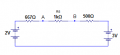# Calculating voltages

#### Transatlantic

Joined Feb 6, 2014
44
Given a circuit like this :Can you figure out what the voltages are (even just roughly) by just inpection? as opposed to using a nodal analysis or a simulation solution?

By inspection I mean applying simples rules for series/parallel resistors and voltages dividers, and coming to a rough conclusion in your head.

I was trying to figure out what the voltage would be on R1 or R4. But kept getting in loops, as the resistance for the left divider affects the resistance for the right divider and vice versa.

#### Jony130

Joined Feb 17, 2009
5,457
Does the Thevenin's is allowed ?
1 - remove R5 and find Va and Vb just by using voltage divider formula
Va = 6*1/3 = 2V; Vb = 6*1/2 = 3V
2 - Rth1 = R3||R1 = 2kΩ/3 = 667Ω ; Rth2 = R3||R3 = 1kΩ/2 = 500Ω
And the circuit will now look like thisAnd the current is

I = (3V - 2V)/(0.667kΩ + 1kΩ + 0.5kΩ) ≈ 1V/2.167kΩ ≈ 0.46mA ≈ 460μA. And finally the Va and Vb voltage are equal to:
Va ≈ 3V - (0.46mA*1.5kΩ ) ≈ 2.31V
Vb ≈ 3V - (0.46mA * 0.5kΩ) ≈ 2.77V

Last edited:

#### Transatlantic

Joined Feb 6, 2014
44
I dont understand what you just did. The only examples I've done with Thevenin theorm, have been really simple.

I'll have to readup on it again.

#### MrAl

Joined Jun 17, 2014
10,599
Hello,

A rough idea we could try would be as follows (working in K ohms)...

First we see that VA is less than VB.

That means current flow is right to left in R5.
VA=2v, VB=3v, by inspection with R5=+infinity.
R1 and R3 in parallel, R13, is 2/3 by inspection
R2 and R4 in parallel, R24, is 1/2 by inspection.

If VA was really 2 and VB was really 3, then the current through R5 would be 1ma.
If that were a current source, it would influence VA by one half of +1ma*R13
and influence VB by one half of -1ma*R24.
That means:
VA=2+1/3=2.33v
VB=3-1/4=2.75v

as approximations.

To take this one step further, we could try using these two new voltages to get the
next estimate which should come out closer, if we felt we really needed that.

BTW to parallel two resistors that are not of equal value but one is an integer multiple of the other, find out how many of the higher value it would take to make up one of the lower values, then put them all in parallel, then divide by the total number.
For example, to parallel 3k and 1k, we know that three 3k resistors in parallel makes up 1k, so that means we have four 3k resistors in total in parallel, which means we have 3/4 k ohms total.

Last edited:

#### #12

Joined Nov 30, 2010
18,224
Can you figure out what the voltages are (even just roughly) by just inpection? as opposed to using a nodal analysis or a simulation solution?
2v on the left junction, 3V on the right junction, except they are smeared towards each other by a similar impedance in the middle.
So, significantly more than 2v on the left junction and significantly less than 3 v on the right junction.

#### crutschow

Joined Mar 14, 2008
32,896
2v on the left junction, 3V on the right junction, except they are smeared towards each other by a similar impedance in the middle.
So, significantly more than 2v on the left junction and significantly less than 3 v on the right junction.
Following along on that line of reasoning:
So, by some head math, the voltage at point A is Va = 2V and the voltage at point B is Vb = 3V without R5 connected.
More head math shows that the equivalent impedance at point A is some over 500Ω and the equivalent resistance at point B is 500Ω, giving an equivalent total resistance of some over 1kΩ for A and B in series.
Thus the addition of the 1kΩ resistor R5 will cause a voltage drop of about 1/2 the difference between Va and Vb or 1/2V.
This drop is divided roughly between points A and B, so point A is about 1/4 above the difference between Va and Vb, and the voltage at point B will be about 1/4 below the difference between Va and Vb, i.e. A ≅ 2¼V and B ≅ 2¾V.
The calculated (simulated) values are A = 2.31V and B = 2.77V.